# SOLVING INEQUALITIES Solve Then graph the solution Solve

• Slides: 32SOLVING INEQUALITIESSolve. Then graph the solution.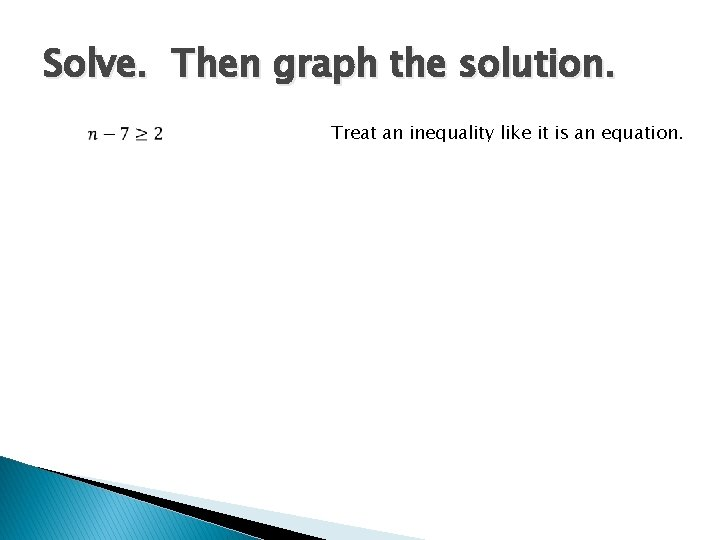Solve. Then graph the solution. Treat an inequality like it is an equation.Solve. Then graph the solution. Treat an inequality like it is an equation.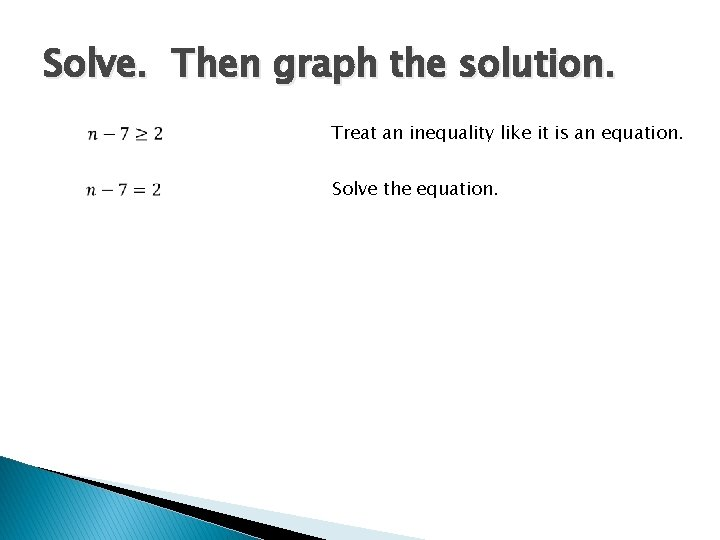Solve. Then graph the solution. Treat an inequality like it is an equation. Solve the equation.Solve. Then graph the solution. Treat an inequality like it is an equation. Solve the equation.Solve. Then graph the solution. Treat an inequality like it is an equation. Solve the equation. Reinsert the inequality symbol.Solve. Then graph the solution. Treat an inequality like it is an equation. Solve the equation. Reinsert the inequality symbol.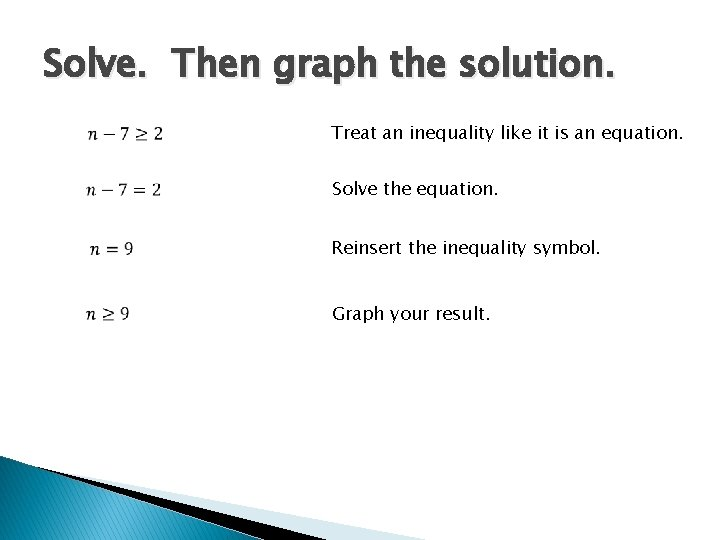Solve. Then graph the solution. Treat an inequality like it is an equation. Solve the equation. Reinsert the inequality symbol. Graph your result.Solve. Then graph the solution. Treat an inequality like it is an equation. Solve the equation. Reinsert the inequality symbol. Graph your result. 9Solve. Then graph the solution. Treat an inequality like it is an equation. Solve the equation. Reinsert the inequality symbol. Graph your result. 9Solve. Then graph the solution. Treat an inequality like it is an equation. Solve the equation. Reinsert the inequality symbol. Graph your result. 9Solve. Then graph the solution. Treat an inequality like it is an equation. Solve the equation. Reinsert the inequality symbol. Graph your result. 9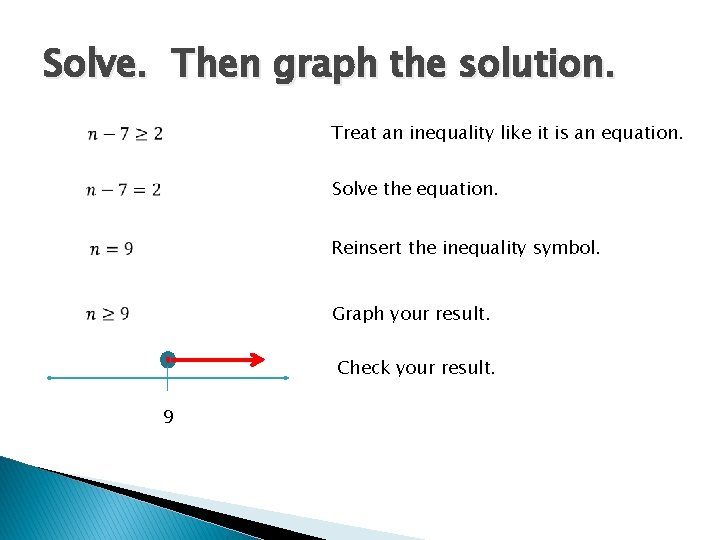Solve. Then graph the solution. Treat an inequality like it is an equation. Solve the equation. Reinsert the inequality symbol. Graph your result. Check your result. 9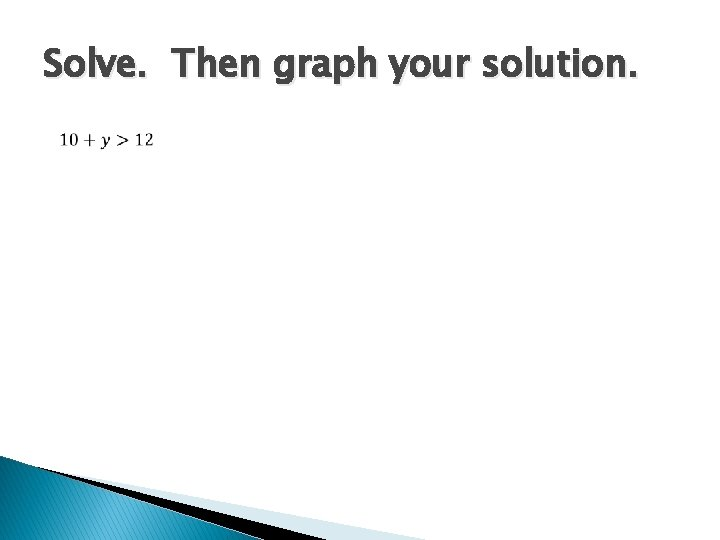Solve. Then graph your solution.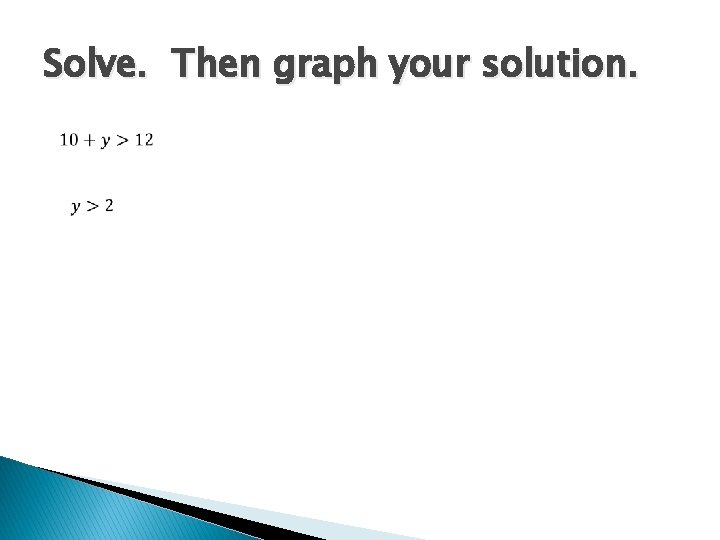Solve. Then graph your solution.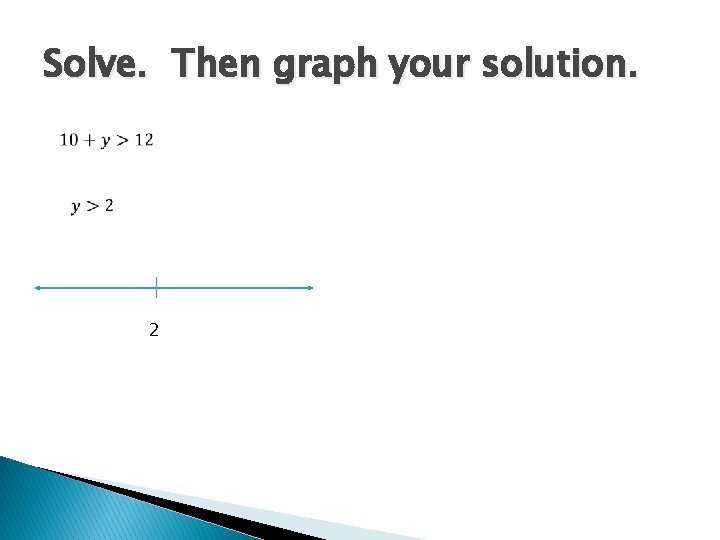Solve. Then graph your solution. 2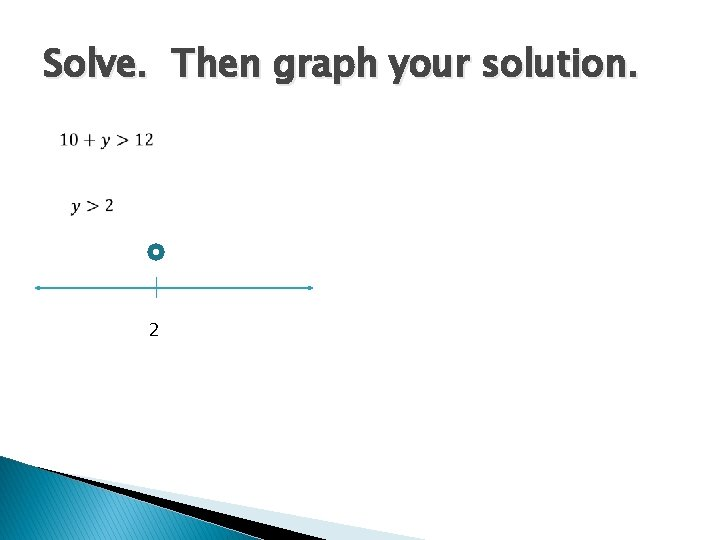Solve. Then graph your solution. 2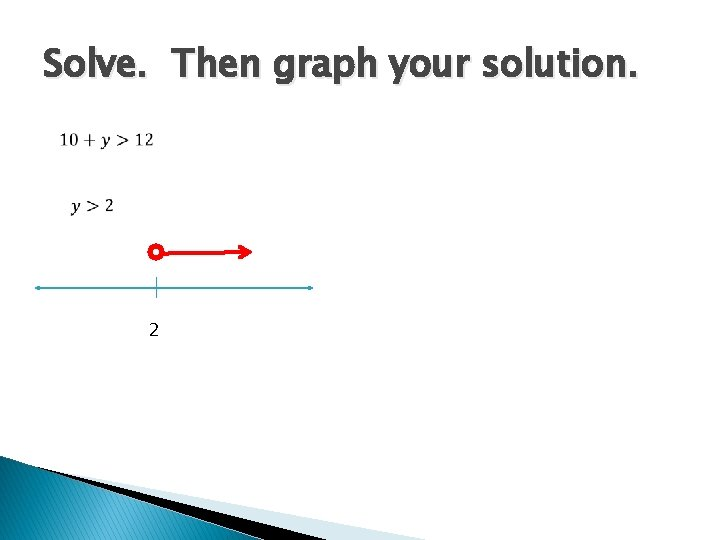Solve. Then graph your solution. 2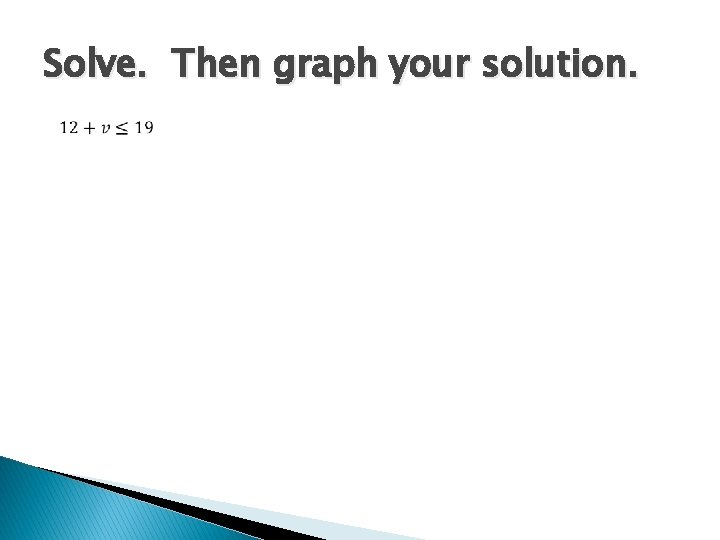Solve. Then graph your solution.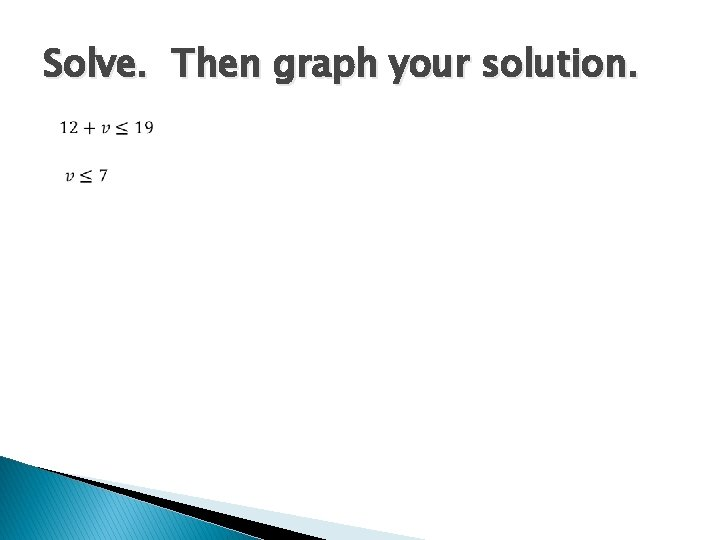Solve. Then graph your solution.Solve. Then graph your solution. 7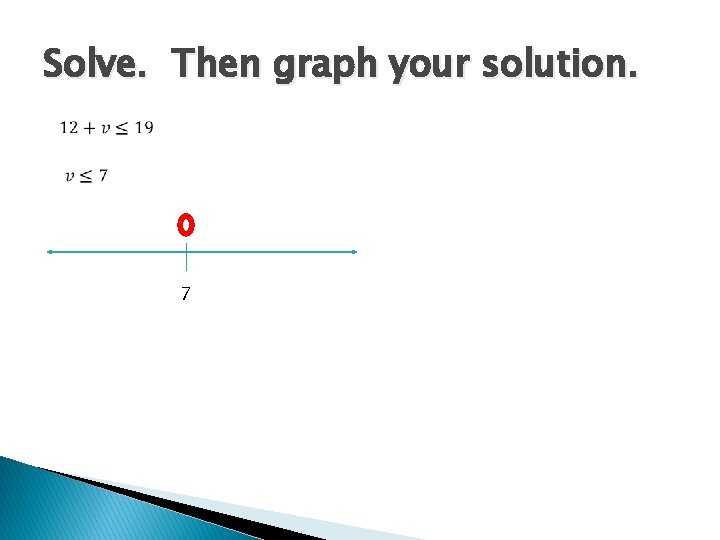Solve. Then graph your solution. 7Solve. Then graph your solution. 7Solve. Then graph your solution. 7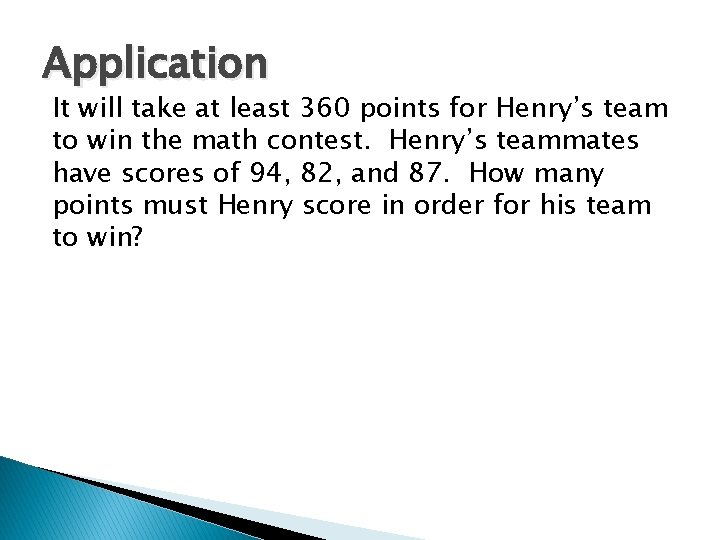Application It will take at least 360 points for Henry’s team to win the math contest. Henry’s teammates have scores of 94, 82, and 87. How many points must Henry score in order for his team to win?Application It will take at least 360 points for Henry’s team to win the math contest. Henry’s teammates have scores of 94, 82, and 87. How many points must Henry score in order for his team to win?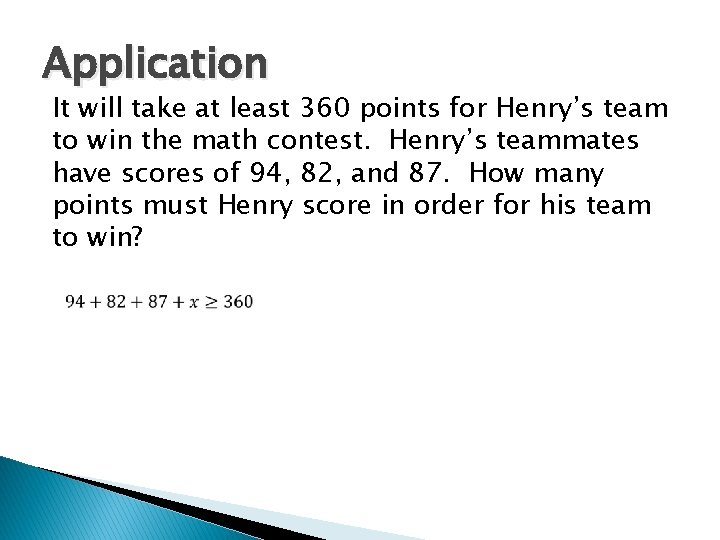Application It will take at least 360 points for Henry’s team to win the math contest. Henry’s teammates have scores of 94, 82, and 87. How many points must Henry score in order for his team to win?Application It will take at least 360 points for Henry’s team to win the math contest. Henry’s teammates have scores of 94, 82, and 87. How many points must Henry score in order for his team to win?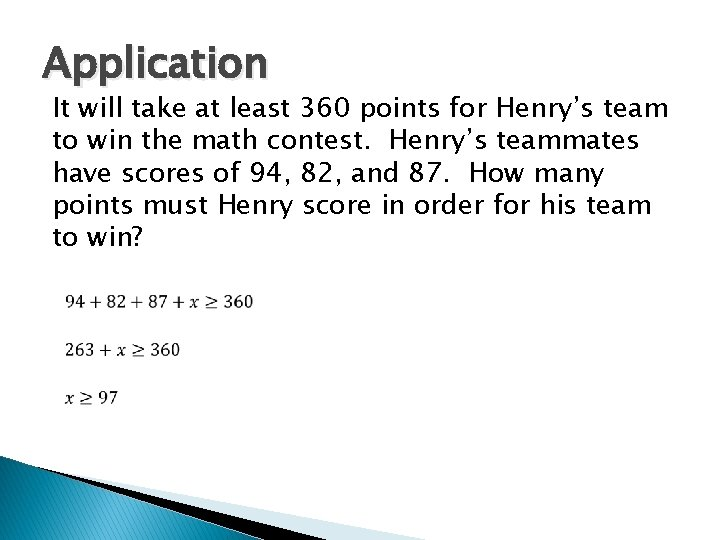Application It will take at least 360 points for Henry’s team to win the math contest. Henry’s teammates have scores of 94, 82, and 87. How many points must Henry score in order for his team to win?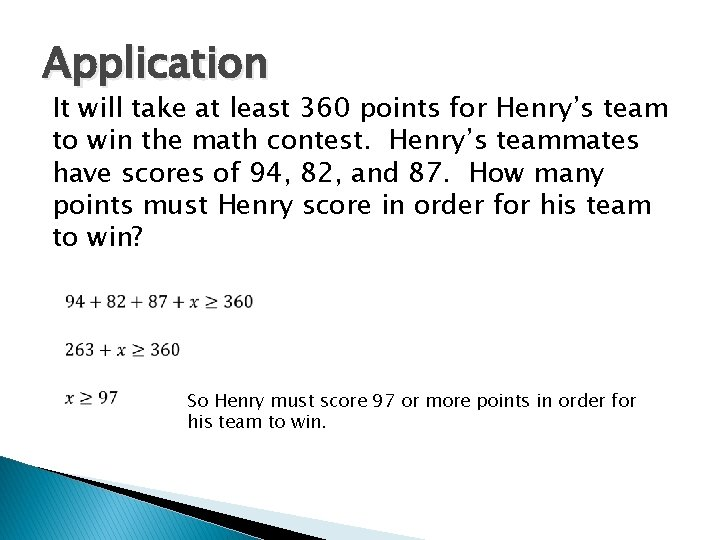Application It will take at least 360 points for Henry’s team to win the math contest. Henry’s teammates have scores of 94, 82, and 87. How many points must Henry score in order for his team to win? So Henry must score 97 or more points in order for his team to win.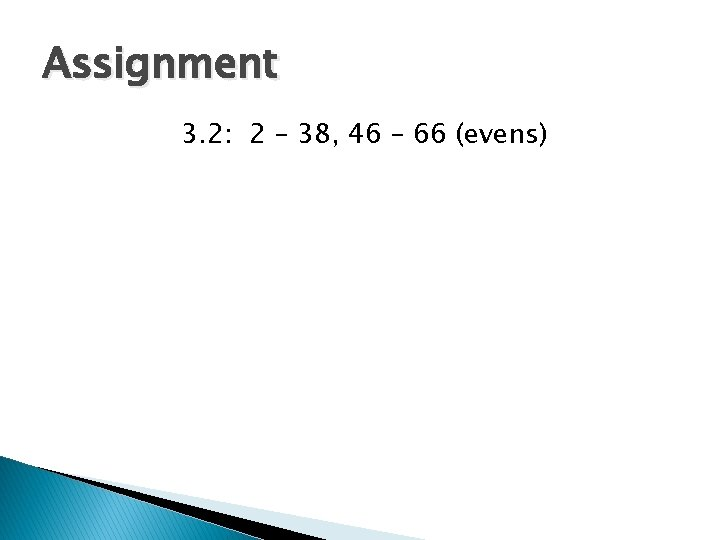Assignment 3. 2: 2 – 38, 46 – 66 (evens)